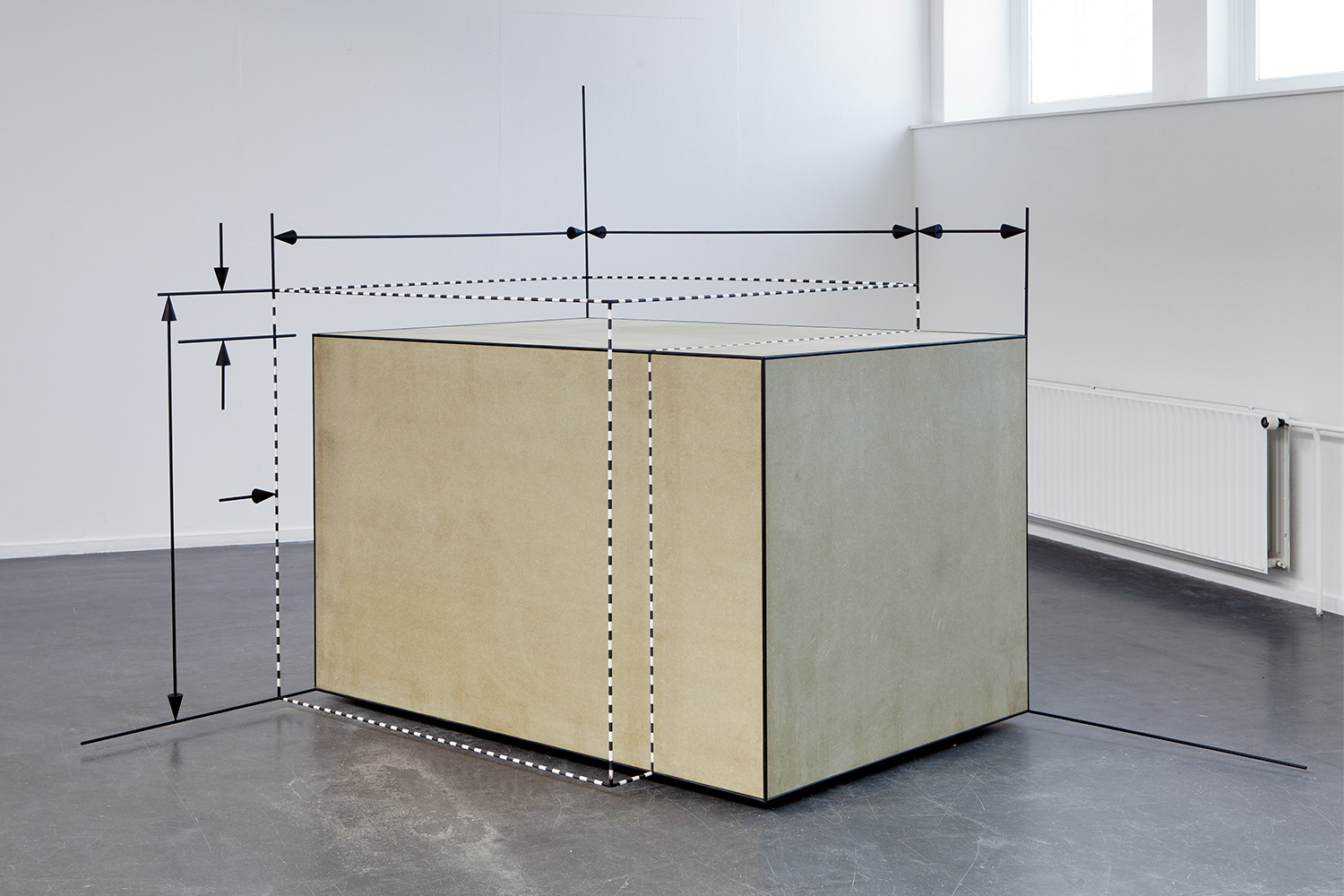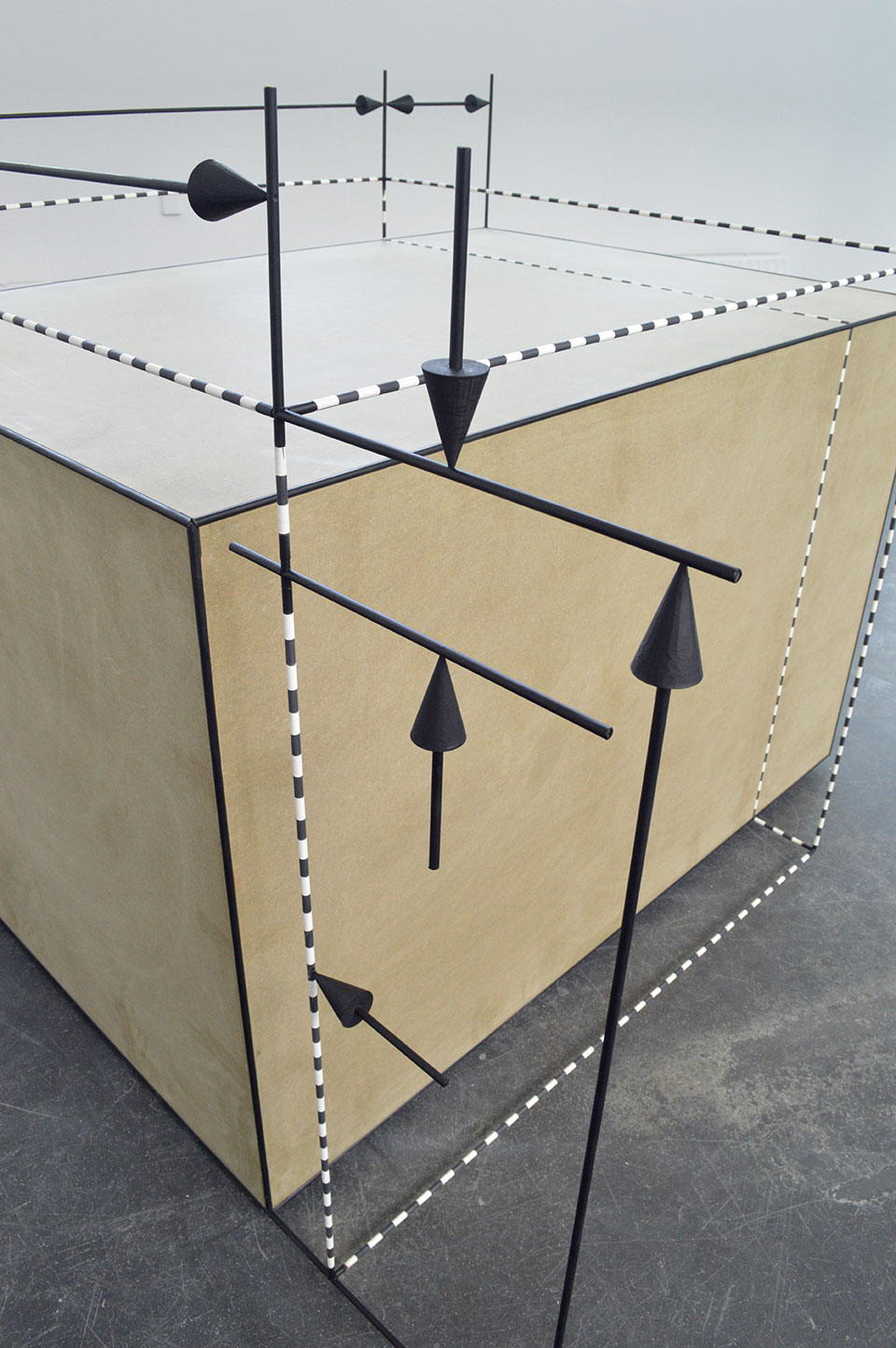rick van meel | work | news | about Change of Shape of an Element in Tension | 2017 | mdf, steel, plastic | 203 x 253 x 189 cm"Change of Shape of an Element in Tension." Because the dimensions of a bar in tension or compression are changed when a load is applied, the volume of the bar changes too. The change in volume can be calculated from the axial and lateral strains. Let us take a small element of isotropic material cut from a bar in tension. The original shape of the element is a cube having sides of lengths a, b, and c in the x, y, and z directions, respectively. The x axis is taken in the longitudinal direction of the bar, which is the direction of the normal stresses σ produced by the axial forces. The elongation of the element in the direction of loading is a∈, where ∈ is the axial strain. Because the lateral strains are - v∈, the lateral dimensions decrease by bv∈ and cv∈ in the y and z directions, respectively. Thus the final dimensions of the element are a(1 + ∈), b(1 - v∈), and c(1 - v∈), and the final volume is Vş = abc(1 + ∈) (1 - v∈) (1 - v∈) * Gere, J.M. en Timoshenko, S. P. (1991) Mechanics of materials (3e SI ed.) 1. A point is that which has no part. 2. A line is breadthless length. 3. The ends of a line are points. 4. A straight line is a line which lies evenly with the points on itself. 5. A surface is that which has length and breadth only. 6. The edges of a surface are lines. 7. A plane surface is a surface which lies evenly with the straight lines on itself. 8. A plane angle is the inclination to one another of two lines in a plane which meet one another and do not lie in a straight line. 9. And when the lines containing the angle are straight, the angle is called rectilinear. 10. When a straight line standing on a straight line makes the adjacent angles equal to one another, each of the equal angles is right, and the straight line standing on the other is called a perpendicular to that on which it stands. 11. An obtuse angle is an angle greater than a right angle. 12. An acute angle is an angle less than a right angle. 13. A boundary is that which is an extremity of anything. 14. A figure is that which is contained by any boundary or boundaries. Et cetera.* Byrne, O. (2013) The Elements of Euclid (1e ed.) ^